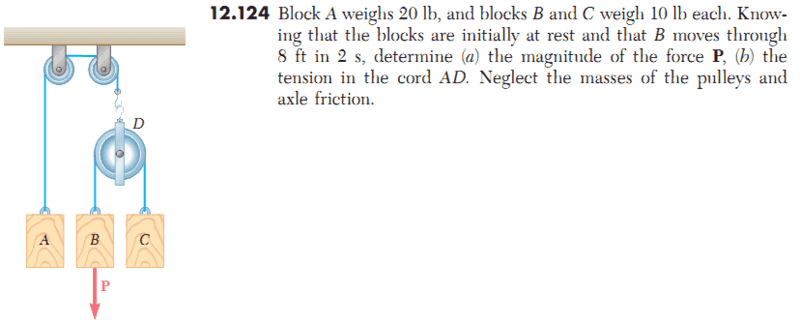# Dynamics of a particle

HWGXX7

## Homework Statement## Homework Equations

I converted the given data to SI units, because of my native calculation system.
I used an index for particles and named rope force BC: $$T_{BC}$$

## The Attempt at a Solution

Kinematic expression for particle B:
$$a=constant=1,22 \frac{m}{s^{2}}$$
$$v=a.t$$
$$x(2)=a.\frac{t^{2}}{2}=2,44 m$$

Dynamic expression for particle B:
$$m_{B}.a_{B}=44,5+P-T_{BC} => 4,54 kg.1,22\frac{m}{s^{2}}= 44,5+P-T_{BC}$$

Finding unknown rope force BC form dynamic expression of particle C:
Acceleration of particle C is the same as particle B
$$-5,54=44,5-T_{BC}$$
$$T_{BC}=-50,.04N$$

Apply this in equation for particle B gives: $$5,54=44,5+P-50,04 => P=11,08N$$

(Correct) solution has to be: 7,37 N

What did I do wrong?

Mentor
Notice that your solution doesn't include any effects from body A. That should make you suspiciousIf pulley D accelerates downwards (as body A accelerates upwards), then this will affect the tension you find. If the pulley is accelerating downward then the whole "subsystem" consisting of the pulley with body B and body C will share the same net acceleration. One effect of this will be to reduce the effective gravitational acceleration acting on B and C. If we call the downward acceleration of the pulley A1, then the effective gravitational acceleration operating on B and C will be g - A1.

HWGXX7
I reviewed the problem and came to follow set of equations:

Subsystem with particle B and C (and the pully) has an acceleration $$a_{1}$$
Newton second law:
$$+9,08.a_{1}=+P+89-T_{AD}$$

Particle A move with same acceleration upward, Newton second law:
$$-9,08.a_{1}=+89-T_{AD}$$

Substituting the equations gives me: $$2.T_{AD}=178+P$$

Now I do have to find a relation between the acceleration of the subsystem and particle B.
I know that the effective acceleration of particle B is:$$1,22 m/s^{2}$$.
But I don't understand why $$a_{1}$$ equals $$9,81 m/s^{2}-1,22m/s^{2}$$

Could you explain this to me?

Mentor
I reviewed the problem and came to follow set of equations:

Subsystem with particle B and C (and the pully) has an acceleration $$a_{1}$$
Newton second law:
$$+9,08.a_{1}=+P+89-T_{AD}$$

Particle A move with same acceleration upward, Newton second law:
$$-9,08.a_{1}=+89-T_{AD}$$

Substituting the equations gives me: $$2.T_{AD}=178+P$$

Now I do have to find a relation between the acceleration of the subsystem and particle B.
I know that the effective acceleration of particle B is:$$1,22 m/s^{2}$$.
But I don't understand why $$a_{1}$$ equals $$9,81 m/s^{2}-1,22m/s^{2}$$

Could you explain this to me?

I don't think I can explain it, because I don't think that it's true if I'm correctly understanding that "9,81" is g and "1,22" is the net acceleration of block BI believe that the acceleration a1 of the subsystem as a whole (and hence block A also) should be closer to 0.4 m/s2.

What sort of "relation between the acceleration of the subsystem and particle B" are you looking for and why? Didn't already solve for force P?

HWGXX7
Here are my usefull equation to solve problem:
$$2.T_{AD}=178+P$$
$$9,08.a_{1}=P+89-T_{AD}$$
The acceleration of particle B consist of 2 components:
$$a_{1}=a_{pully}$$
$$a_{Beffectif}=1,22 m/s^{2}=a_{1}+a_{particle B}$$
$$m_{particle B}.a_{particle B}=P+44,5-T_{BC}$$

Particle C will accelerat upwards because of force P and the subsystem will move down.

With this configuration I still cannot solve it..

By the way the solution you proposed is indeed correct.

grtz

HWGXX7
Oke, I think I got the correct appoarch with the relevant assumptions according to the kinematics. It was actualy a bit more tricky than just putting in some variabeles.

I will write the solution-equation in the assumption the system is moving:

- particle A: $$-m_{A}.a_{A}=F_{g,A}-T_{AD}$$
- subsystem (containing pully D en particle B and C): $$+(m_{B}+m_{C}).a_{A}-T_{AD}+P$$

This lead to: $$178-2.T_{AD}+P=0$$
$$18,14.a_{A}=P$$

Now the tricky part I first looked over: the given kinematic expression of particle B is abolute. This means that: $$1,44m/s^{2}=a_{B/D}+a_{D}$$

For particle C:$$a_{C}=a_{D}-a_{C/D}$$
$$a_{C/D}=a_{D}-a_{C}$$

We know that: $$a_{C/D}=a_{B/D}$$
The dynamics of particle C: $$a_{C}.m_{C}=+44,5-T_{BC}$$
$$T_{BC}=44,5+m_{A}.(a_{D}-a_{C/D})$$
So now I can write rope force BC as function of acceleration:
$$T_{BC}=44,5+m_{A}.(a_{D}-a_{A/D})$$

The dynamics of particel B: $$m_{B}.a_{B}=+P+44,5-T_{BC}=6,5N$$
$$m_{B}.a_{B}=+18,14.a_{A}+44,5-T_{BC}=6,5N$$
$$m_{B}.a_{B}=+18,14.(1,44-a_{B/D})+44,5-T_{BC}=6,5N$$

Putting this all together get's me: $$a_{D}=0,479 m/s^{2}$$

Still a bit incorrect, but more important to me is the method. So my final question are the assumptions I used correct?

grtz

Mentor
Your assumptions and approach look okay to me.

Personally, I like to leave everything in symbolic form for as long as possible in order to avoid having to "carry around" a lot of numbers through a derivation and to avoid accumulating rounding errors.

HWGXX7
Personally, I like to leave everything in symbolic form for as long as possible in order to avoid having to "carry around" a lot of numbers through a derivation and to avoid accumulating rounding errors.

Indeed, but I wanted to make it clear that I got the good approach. So with a couple of number it's more easily.

Offcourse the errors occurred at my final solution is due to the fact of rounding solution at the steps I took.

thank for all the help!